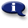# .

## Syllabus Information

Winter 2022
Oct 01,2022Use this page to maintain syllabus information, learning objectives, required materials, and technical requirements for the course.

Syllabus Information
MTH 010 - Whole Numbers, Fractions, Decimals
Associated Term: Winter 2022
Learning Objectives: Upon successful completion of this course, the student should be able to: 1. Add, subtract, multiply, and divide whole numbers 2. Identify characteristics of even, odd, prime, and composite numbers 3. Solve real world application problems using whole numbers 4. Order whole numbers using < and > 5. List factors and multiples of a given number 6. Compute problems using the order of operations 7. Use math vocabulary 8. Compute area and perimeter of rectangles using whole numbers 9. Add, subtract, multiply, and divide fractions with like and unlike denominators 10. Reduce fractions 11. Compare fractions using <, > or = 12. Convert fractions to decimals 13. Solve real world problems using fractions 14. Compute area and perimeter of rectangles using fractions 15. Use vocabulary of fraction terms 16. Add, subtract, multiply, and divide using decimals 17. Identify place value in decimal numbers 18. Compare decimals using <, > or = 19. Convert decimals to fractions 20. Solve real world application problems using decimals 21. Compute area and perimeter of rectangles using decimals 22. Selects appropriate math study strategies 23. Monitors and evaluates personal confidence progress 24. Utilizes appropriate math resources
Required Materials:
Technical Requirements: